# Koch Curve or Koch Snowflake

• Last Updated : 03 Oct, 2018

What is Koch Curve?

The Koch snowflake (also known as the Koch curve, Koch star, or Koch island) is a mathematical curve and one of the earliest fractal curves to have been described. It is based on the Koch curve, which appeared in a 1904 paper titled “On a continuous curve without tangents, constructible from elementary geometry” by the Swedish mathematician Helge von Koch.

Attention geek! Strengthen your foundations with the Python Programming Foundation Course and learn the basics.

To begin with, your interview preparations Enhance your Data Structures concepts with the Python DS Course. And to begin with your Machine Learning Journey, join the Machine Learning - Basic Level Course

The progression for the area of the snowflake converges to 8/5 times the area of the original triangle, while the progression for the snowflake’s perimeter diverges to infinity. Consequently, the snowflake has a finite area bounded by an infinitely long line.

# Construction

Step1:

Draw an equilateral triangle. You can draw it with a compass or protractor, or just eyeball it if you don’t want to spend too much time drawing the snowflake.

• It’s best if the length of the sides are divisible by 3, because of the nature of this fractal. This will become clear in the next few steps.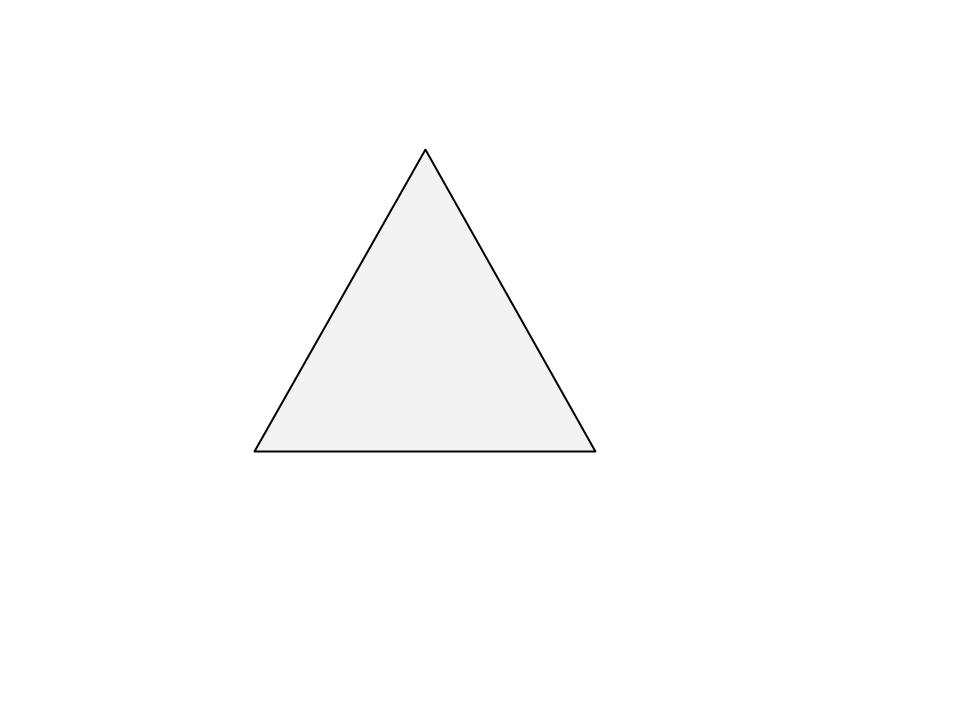Step2:

Divide each side in three equal parts. This is why it is handy to have the sides divisible by three.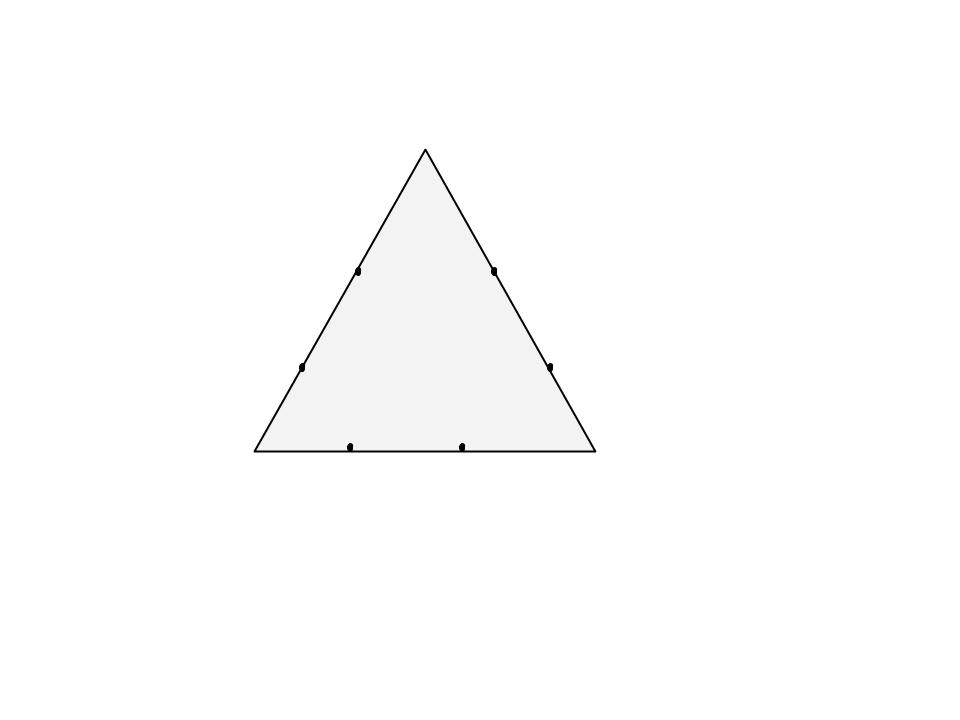Step3:

Draw an equilateral triangle on each middle part. Measure the length of the middle third to know the length of the sides of these new triangles.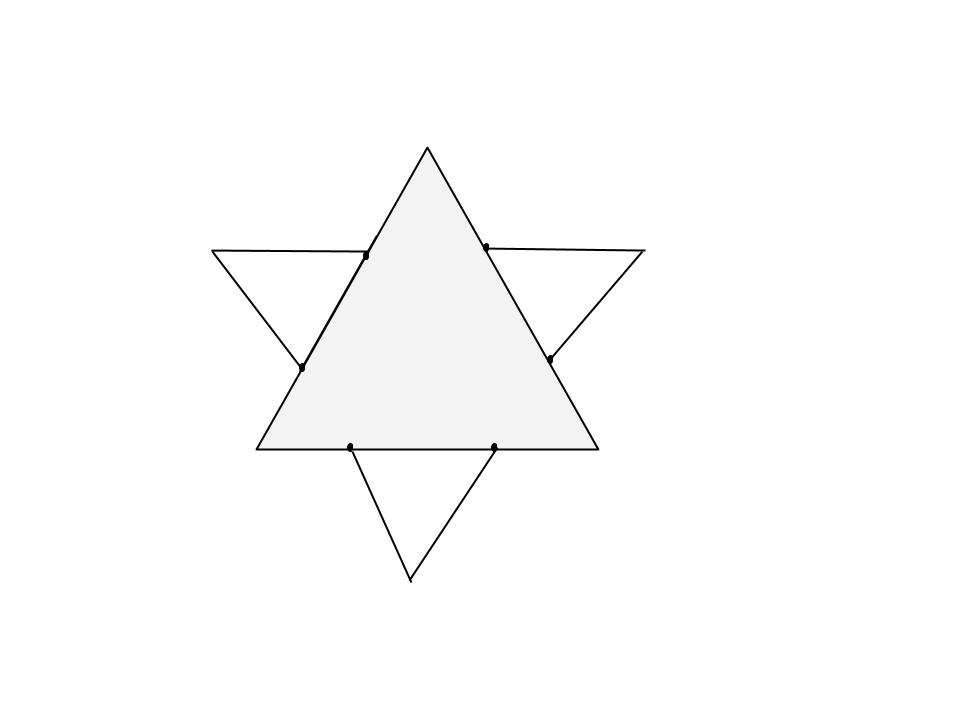Step4:

Divide each outer side into thirds. You can see the 2nd generation of triangles covers a bit of the first. These three line segments shouldn’t be parted in three.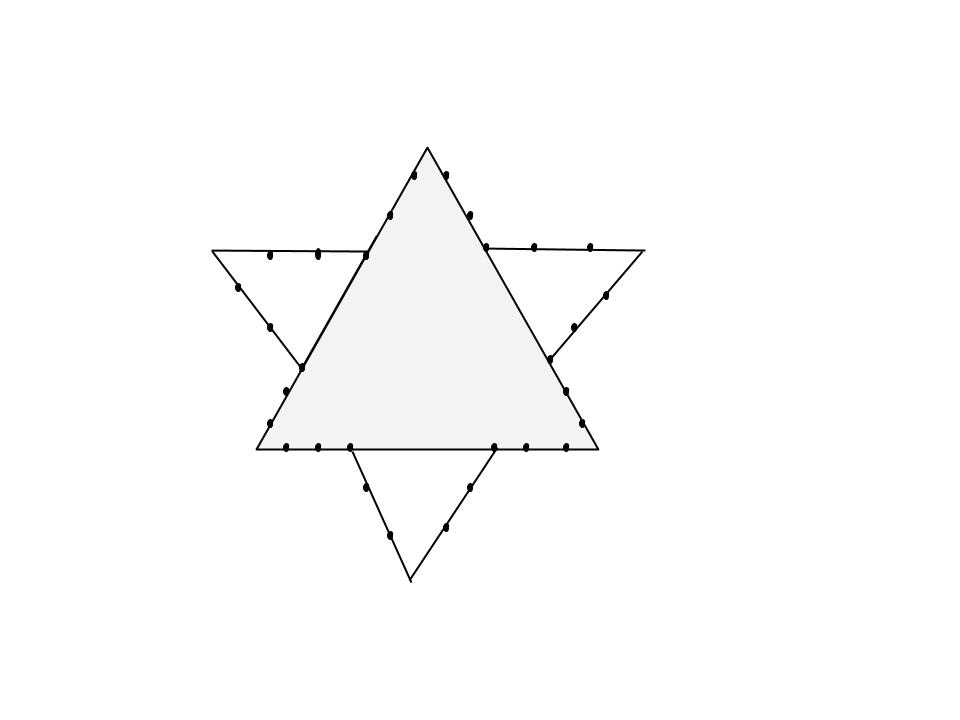Step5:

Draw an equilateral triangle on each middle part.

• Note how you draw each next generation of parts that are one 3rd of the mast one.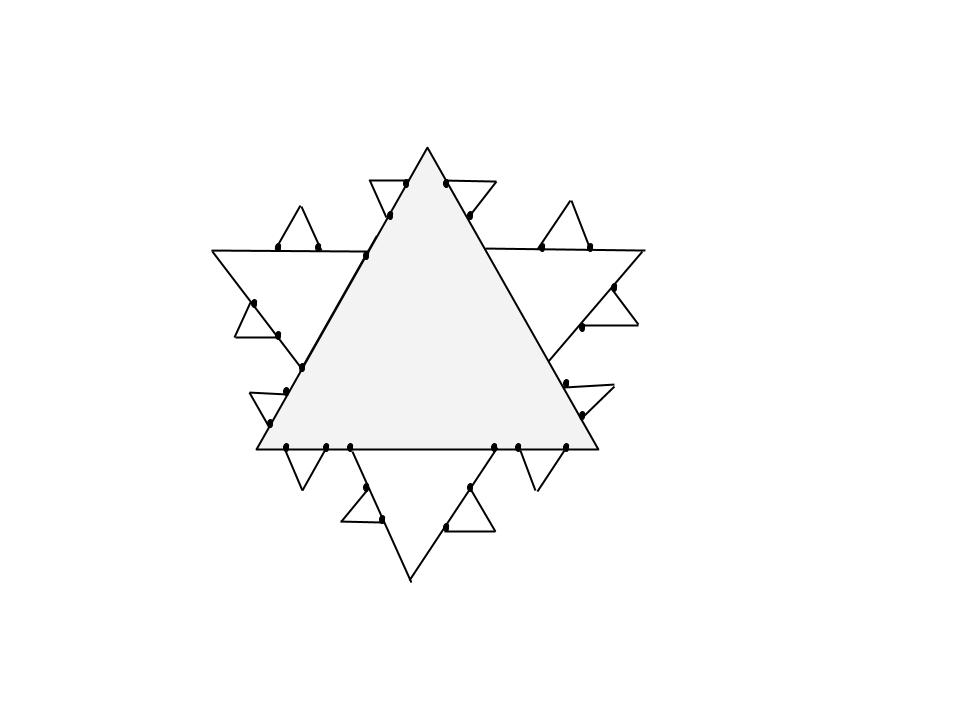# Representation as Lindenmayer system

The Koch curve can be expressed by the following rewrite system (Lindenmayer system):

Alphabet : F
Constants : +, ?
Axiom : F
Production rules: F ? F+F–F+F

Here, F means “draw forward”, – means “turn right 60°”, and + means “turn left 60°”.
To create the Koch snowflake, one would use F++F++F (an equilateral triangle) as the axiom.

# To create a Koch Curve :

 `# Python program to print partial Koch Curve.``# importing the libraries : turtle standard ``# graphics library for python``from` `turtle ``import` `*`` ` `#function to create koch snowflake or koch curve``def` `snowflake(lengthSide, levels):``    ``if` `levels ``=``=` `0``:``        ``forward(lengthSide)``        ``return``    ``lengthSide ``/``=` `3.0``    ``snowflake(lengthSide, levels``-``1``)``    ``left(``60``)``    ``snowflake(lengthSide, levels``-``1``)``    ``right(``120``)``    ``snowflake(lengthSide, levels``-``1``)``    ``left(``60``)``    ``snowflake(lengthSide, levels``-``1``)`` ` `# main function``if` `__name__ ``=``=` `"__main__"``:`` ` `    ``# defining the speed of the turtle``    ``speed(``0``)                   ``    ``length ``=` `300.0`              ` ` `    ``# Pull the pen up – no drawing when moving.``    ``penup()                     ``     ` `    ``# Move the turtle backward by distance, ``    ``# opposite to the direction the turtle ``    ``# is headed.``    ``# Do not change the turtle’s heading.``    ``backward(length``/``2.0``)        `` ` `    ``# Pull the pen down – drawing when moving.``    ``pendown()         `` ` `    ``snowflake(length, ``4``)`` ` `    ``# To control the closing windows of the turtle``    ``mainloop() `

Output:

To create a full snowflake with Koch curve, we need to repeat the same pattern three times. So lets try that out.

 `# Python program to print complete Koch Curve.``from` `turtle ``import` `*`` ` `# function to create koch snowflake or koch curve``def` `snowflake(lengthSide, levels):``    ``if` `levels ``=``=` `0``:``        ``forward(lengthSide)``        ``return``    ``lengthSide ``/``=` `3.0``    ``snowflake(lengthSide, levels``-``1``)``    ``left(``60``)``    ``snowflake(lengthSide, levels``-``1``)``    ``right(``120``)``    ``snowflake(lengthSide, levels``-``1``)``    ``left(``60``)``    ``snowflake(lengthSide, levels``-``1``)`` ` `# main function``if` `__name__ ``=``=` `"__main__"``:``    ``# defining the speed of the turtle``    ``speed(``0``)                   ``    ``length ``=` `300.0`   ` ` `    ``# Pull the pen up – no drawing when moving.``    ``# Move the turtle backward by distance, opposite``    ``# to the direction the turtle is headed.``    ``# Do not change the turtle’s heading.           ``    ``penup()                     `` ` `    ``backward(length``/``2.0``)`` ` `    ``# Pull the pen down – drawing when moving.        ``    ``pendown()           ``    ``for` `i ``in` `range``(``3``):    ``        ``snowflake(length, ``4``)``        ``right(``120``)`` ` `     ``# To control the closing windows of the turtle``    ``mainloop()       `

Output:

```https://media.geeksforgeeks.org/wp-content/uploads/output_2.mp4
```

This article is contributed by Subhajit Saha. If you like GeeksforGeeks and would like to contribute, you can also write an article using contribute.geeksforgeeks.org or mail your article to contribute@geeksforgeeks.org. See your article appearing on the GeeksforGeeks main page and help other Geeks.